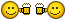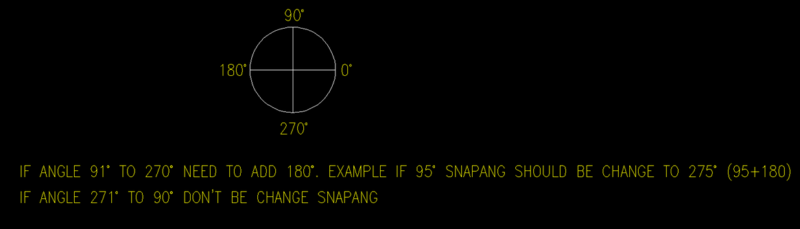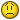# Setvar 'Snapang using lisp with greater-than or equal condition

## Recommended Posts```(defun RtoD (nbrOfRadians)
)

(defun DtoR (nbrOfDegrees)
(* (/ nbrOfDegrees 180.0) pi)
)

(if (>= (cvunit (getvar 'snapang) "radians" "degrees") 180.0)
(setvar 'snapang (- (cvunit (getvar 'snapang) "radians" "degrees") 180.0))
)```

Edited by Pugazh

##### Share on other sites```(defun RtoD (nbrOfRadians)
)

(defun DtoR (nbrOfDegrees)
(* (/ nbrOfDegrees 180.0) pi)
)

(if (>= (cvunit (getvar 'snapang) "radians" "degrees") 180.0)
(command "_.snapang" (- (cvunit (getvar 'snapang) "radians" "degrees") 180.0))
)```

This code is working fine##### Share on other sitesThe code could alternatively be written:

```(if (<= pi (getvar 'snapang))
(setvar 'snapang (- (getvar 'snapang) pi))
)```

Or simply:

`(setvar 'snapang (rem (getvar 'snapang) pi))`

•2

##### Share on other sitesthanks @Lee MacEdited by Pugazh

##### Share on other sitesHi @Lee Mac,

i want as per attached image have two condition in one lisp code it's possible? (must be check the two condition at the same time)##### Share on other sites`(if (< (/ pi 2) (getvar 'snapang) (* (/ pi 2) 3)) (setvar 'snapang (+ (getvar 'snapang) pi)))`

•1

##### Share on other sitesAlternatively, without if statements:

`(setvar 'snapang (- (rem (+ (getvar 'snapang) (/ pi 2.0)) pi) (/ pi 2.0)))`

•1
•1

##### Share on other sitesWow !! Exactly  i needed this onethank you @dlanorh & @Lee Mac cheers##### Share on other sitesHow to combine ? one if condition```(if (< (/ pi 2) (getvar 'snapang) (* (/ pi 2) 3)) (setvar 'snapang (+ (getvar 'snapang) pi)))
(if (= (getvar 'snapang) (/ pi 2)) (setvar 'snapang (/ pi 2)))
(if (= (getvar 'snapang) (* (/ pi 2) 3)) (setvar 'snapang (/ pi 2)))
```

##### Share on other sites`(if (= (setvar 'snapang (- (rem (+ (getvar 'snapang) (/ pi 2.0)) pi) (/ pi 2.0)))(* (/ pi 2) -1))(setvar 'snapang (/ pi 2)))`

Now it works!

Edited by tombu
coding error
•1

##### Share on other sites14 hours ago, tombu said:
```
(if (= (setvar 'snapang (- (rem (+ (getvar 'snapang) (/ pi 2.0)) pi) (/ pi 2.0)))(* (/ pi 2) -1))(setvar 'snapang (/ pi 2)))```

Now it works!

thank you @tombu##### Share on other sitesI have another doubt!

If i set snapang any angle, it should be automatically change to as per autocad dimension text rotation. it's possible ?

##### Share on other sitesAs I do Civil / Survey drawings whose plan views are all twisted in each viewport I wrote this to set selected block insert, text, mtext, & dimtext angles Horizontal.

I've modified it for you to use the snap angle if the view isn't twisted.

```;| ;| https://forums.augi.com/showthread.php?171018-rotate-text-to-horizontal-in-viewport&p=1332928&viewfull=1#post1332928
http://www.theswamp.org/index.php?topic=55060.msg595136#msg595136
Set block insert, text, mtext, & dimtext angle Horizontal by Tom Beauford
Command line: (load "TextHoriz.lsp") TextHoriz |;
(defun c:TextHoriz (/ ss1 num cnt viewtwist snapang etype obj dimr)
(setq ss1 (ssget '((0 . "insert,mtext,text,dimension")))
num (sslength ss1)
cnt 0
viewtwist (getvar "viewtwist")
snapang (- (rem (+ (getvar 'snapang) (/ pi 2.0)) pi) (/ pi 2.0))
)
(if (or (= snapang (* (/ pi 2) -1)) (= snapang (* (/ pi 2) 3)))(setvar 'snapang (/ pi 2)))
(if(/= viewtwist 0.0)
(repeat num
(setq ent (ssname ss1 cnt)etype (cdr(assoc 0 (entget ent))))
(setq obj (vlax-ename->vla-object ent))
(if(= etype "DIMENSION")
(progn
(setq dimr (- (vla-get-Rotation obj)viewtwist))
(vla-put-TextRotation obj dimr)
)
(vla-put-Rotation obj (- viewtwist))
)
(setq cnt (1+ cnt))
) ; repeat
(repeat num
(setq ent (ssname ss1 cnt)etype (cdr(assoc 0 (entget ent))))
(setq obj (vlax-ename->vla-object ent))
(if(= etype "DIMENSION")
(progn
(setq dimr (+ (vla-get-Rotation obj)snapang))
(vla-put-TextRotation obj dimr)
)
(vla-put-Rotation obj snapang)
)
(setq cnt (1+ cnt))
) ; repeat
;	(princ "\nNo TWist in Viewport.")
) ; if
(princ)
)
```

Edited by tombu
Updated to fix both snapang 270 or -90.
•1

##### Share on other sitesThank you @tombu!!

I think my question is wrong, How to take snap angle in aligned dimensions text rotation ? if i draw dimension text automatically rotated as per snap angle.

i need this snap angle code with if conditions

like this

`(if (= (setvar 'snapang (- (rem (+ (getvar 'snapang) (/ pi 2.0)) pi) (/ pi 2.0)))(* (/ pi 2) -1))(setvar 'snapang (/ pi 2)))`

##### Share on other sites5 minutes ago, Pugazh said:

Thank you @tombu!!

I think my question is wrong, How to take snap angle in aligned dimensions text rotation ? if i draw dimension text automatically rotated as per snap angle.

i need this snap angle code with if conditions

like this

```
(if (= (setvar 'snapang (- (rem (+ (getvar 'snapang) (/ pi 2.0)) pi) (/ pi 2.0)))(* (/ pi 2) -1))(setvar 'snapang (/ pi 2)))```

I modified the code for that 38 minutes ago, have you tried the updated code?

##### Share on other sites```    (command "_.snapang" "_non" pt1 "_non" pt2)
(if (= (setvar 'snapang (- (rem (+ (getvar 'snapang) (/ pi 2.0)) pi) (/ pi 2.0)))(* (/ pi 2) -1))(setvar 'snapang (/ pi 2)))```

@tombu , I did not change rotation.

I have two points, pt1 is block mid point, pt2 is block insert point.

now snapang is 270 from the pt1 & pt2. i use this code sometimes update snapangle 90 but sometimes not updatewhy i know##### Share on other sitesOn 10/29/2019 at 5:04 AM, Pugazh said:

How to combine ? one if conditionI've updated the code to correct snapang of either (* (/ pi 2) 3) or (* (/ pi 2) -1).

Edited by tombu
•1

##### Share on other sites@Pugazh Assuming I've understood what you are looking to achieve, is this program of use to you?

•1

##### Share on other sites10 hours ago, Lee Mac said:

@Pugazh Assuming I've understood what you are looking to achieve, is this program of use to you?

thank you leei got it your code##### Share on other sites7 hours ago, Pugazh said:

thank you leei got it your codeExcellent - you're most welcome•1

## Join the conversation

You can post now and register later. If you have an account, sign in now to post with your account.
Note: Your post will require moderator approval before it will be visible.×   Pasted as rich text.   Paste as plain text instead

Only 75 emoji are allowed.